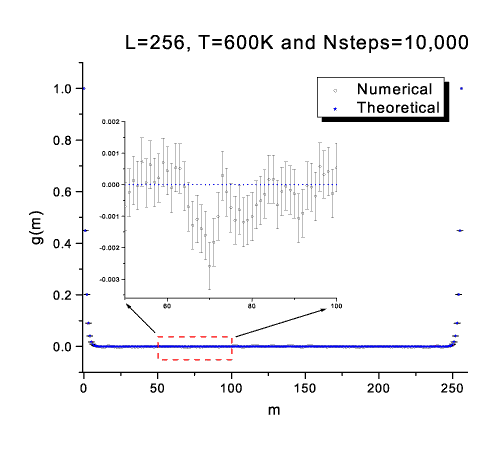Skip Navigation Links.

### Line Symbol Plot of a Monte Carlo SimulationIn the Monte Carlo simulation, it is important to know the spatial correlations between different sites. In this case, they have been calculated for the 1-D Ising Model. This graph shows the comparison between the numerical and theoretical values of the spatial correlations. where L is the total number of sites in the Ising model, T is the temperature, and Nsteps is the number of Monte Carlo steps to execute. All the data were prepared using MATLAB. Then, the result was read into Origin as a ASCII file. I used 'Y Error' to plot the numerical result and 'symbol' for the theoretical result. Finally, I used the command 'Tools/Layer' to add one more layer to clearly show the comparison. The 'Arrow Tool' and 'Box Tool' are also used to show the zoomed area. Mr. Rui Xu has a B.S. degree from Tsinghua University, P.R.China, and is currently a Ph.D. student in the Mechanical Department at UC Berkeley.